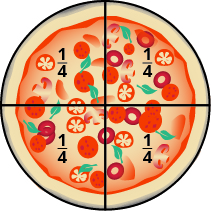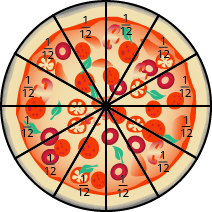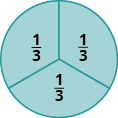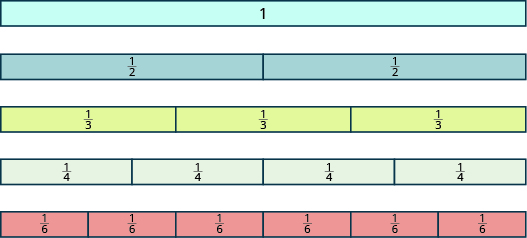## Using Models to Represent Fractions and Mixed Numbers

### Learning Outcomes

• Represent parts of a whole as a fraction with models, numbers, and words
• Identify the numerator and the denominator of a fraction
• Model improper fractions and mixed numbers
• Convert between improper fractions and mixed numbers

### Representing Parts of a Whole as Fractions

Andy and Bobby love pizza. On Monday night, they share a pizza equally. How much of the pizza does each one get? Are you thinking that each boy gets half of the pizza? That’s right. There is one whole pizza, evenly divided into two parts, so each boy gets one of the two equal parts.

In math, we write $\Large{\frac{1}{2}}$ to mean one out of two parts.On Tuesday, Andy and Bobby share a pizza with their parents, Fred and Christy, with each person getting an equal amount of the whole pizza. How much of the pizza does each person get? There is one whole pizza, divided evenly into four equal parts. Each person has one of the four equal parts, so each has $\Large{\frac{1}{4}}$ of the pizza.On Wednesday, the family invites some friends over for a pizza dinner. There are a total of $12$ people. If they share the pizza equally, each person would get $\Large{\frac{1}{12}}$ of the pizza.### Fractions

A fraction is written $\Large{\frac{a}{b}}$, where $a$ and $b$ are integers and $b\ne 0$. In a fraction, $a$ is called the numerator and $b$ is called the denominator.

A fraction is a way to represent parts of a whole. The denominator $b$ represents the number of equal parts the whole has been divided into, and the numerator $a$ represents how many parts are included. The denominator, $b$, cannot equal zero because division by zero is undefined.

In the image below, the circle has been divided into three parts of equal size. Each part represents $\Large{\frac{1}{3}}$ of the circle. This type of model is called a fraction circle. Other shapes, such as rectangles, can also be used to model fractions.Doing the Manipulative Mathematics activity Model Fractions will help you develop a better understanding of fractions, their numerators and denominators.

What does the fraction $\Large{\frac{2}{3}}$ represent? The fraction $\Large{\frac{2}{3}}$ means two of three equal parts.### Example

Name the fraction of the shape that is shaded in each of the figures.Solution:
We need to ask two questions. First, how many equal parts are there? This will be the denominator. Second, of these equal parts, how many are shaded? This will be the numerator.

$\begin{array}{cccc}\text{How many equal parts are there?}\hfill & & & \text{There are eight equal parts}\text{.}\hfill \\ \text{How many are shaded?}\hfill & & & \text{Five parts are shaded}\text{.}\hfill \end{array}$
Five out of eight parts are shaded. Therefore, the fraction of the circle that is shaded is $\Large{\frac{5}{8}}$.

$\begin{array}{cccc}\text{How many equal parts are there?}\hfill & & & \text{There are nine equal parts}\text{.}\hfill \\ \text{How many are shaded?}\hfill & & & \text{Two parts are shaded}\text{.}\hfill \end{array}$
Two out of nine parts are shaded. Therefore, the fraction of the square that is shaded is $\Large{\frac{2}{9}}$.

### Example

Shade $\frac{3}{4}$ of the circle.### Try it

Shade $\Large{\frac{6}{8}}$ of the circle.Shade $\Large{\frac{2}{5}}$ of the rectangle.Watch the following video to see more examples of how to write fractions given a model.

In earlier examples, we used circles and rectangles to model fractions. Fractions can also be modeled as manipulatives called fraction tiles, as shown in the image below. Here, the whole is modeled as one long, undivided rectangular tile. Beneath it are tiles of equal length divided into different numbers of equally sized parts.We’ll be using fraction tiles to discover some basic facts about fractions. Refer to the fraction tiles above to answer the following questions:

 How many $\Large{\frac{1}{2}}$ tiles does it take to make one whole tile? It takes two halves to make a whole, so two out of two is ${\Large\frac{2}{2}}=1$. How many $\Large{\frac{1}{3}}$ tiles does it take to make one whole tile? It takes three thirds, so three out of three is ${\Large{\frac{3}{3}}}=1$. How many $\Large{\frac{1}{4}}$ tiles does it take to make one whole tile? It takes four fourths, so four out of four is ${\Large\frac{4}{4}}=1$. How many $\Large{\frac{1}{6}}$ tiles does it take to make one whole tile? It takes six sixths, so six out of six is ${\Large\frac{6}{6}}=1$. What if the whole were divided into $24$ equal parts? (We have not shown fraction tiles to represent this, but try to visualize it in your mind.) How many $\Large{\frac{1}{24}}$ tiles does it take to make one whole tile? It takes $24$ twenty-fourths, so ${\Large\frac{24}{24}}=1$.

This leads us to the Property of One.

### Property of One

Any number, except zero, divided by itself is one.

${\Large\frac{a}{a}}=1\left(a\ne 0\right)$

### Example

Use fraction circles to make wholes using the following pieces:

1. $4$ fourths
2. $5$ fifths
3. $6$ sixths

### Try it

Use fraction circles to make wholes with the following pieces: $3$ thirds.

Use fraction circles to make wholes with the following pieces: $8$ eighths.

What if we have more fraction pieces than we need for $1$ whole? We’ll look at this in the next example.

### Example

Use fraction circles to make wholes using the following pieces:

1. $3$ halves
2. $8$ fifths
3. $7$ thirds

### try it

Use fraction circles to make wholes with the following pieces: $5$ thirds.

Use fraction circles to make wholes with the following pieces: $5$ halves.

## Model Improper Fractions and Mixed Numbers

In an earlier example, you had eight equal fifth pieces. You used five of them to make one whole, and you had three fifths left over. Let us use fraction notation to show what happened. You had eight pieces, each of them one fifth, ${\Large\frac{1}{5}}$, so altogether you had eight fifths, which we can write as ${\Large\frac{8}{5}}$. The fraction ${\Large\frac{8}{5}}$ is one whole, $1$, plus three fifths, ${\Large\frac{3}{5}}$, or $1{\Large\frac{3}{5}}$, which is read as one and three-fifths.

The number $1{\Large\frac{3}{5}}$ is called a mixed number. A mixed number consists of a whole number and a fraction.

### Mixed Numbers

A mixed number consists of a whole number $a$ and a fraction ${\Large\frac{b}{c}}$ where $c\ne 0$. It is written as follows.

$a{\Large\frac{b}{c}}\text{, }c\ne 0$

Fractions such as ${\Large\frac{5}{4}},{\Large\frac{3}{2}},{\Large\frac{5}{5}}$, and ${\Large\frac{7}{3}}$ are called improper fractions. In an improper fraction, the numerator is greater than or equal to the denominator, so its value is greater than or equal to one. When a fraction has a numerator that is smaller than the denominator, it is called a proper fraction, and its value is less than one. Fractions such as ${\Large\frac{1}{2}},{\Large\frac{3}{7}}$, and ${\Large\frac{11}{18}}$ are proper fractions.

### Proper and Improper Fractions

The fraction ${\Large\frac{a}{b}}$ is a proper fraction if $a<b$ and an improper fraction if $a\ge b$.

### Example

Name the improper fraction modeled. Then write the improper fraction as a mixed number.### Example

Draw a figure to model ${\Large\frac{11}{8}}$.

### Try it

Draw a figure to model ${\Large\frac{7}{6}}$

Draw a figure to model ${\Large\frac{6}{5}}$

### Example

Use a model to rewrite the improper fraction ${\Large\frac{11}{6}}$ as a mixed number.

### Try it

In the next video we show another way to draw a model that represents a fraction.  You will see example of both proper and improper fractions shown.

### Example

Use a model to rewrite the mixed number $1{\Large\frac{4}{5}}$ as an improper fraction.

## Contribute!

Did you have an idea for improving this content? We’d love your input.